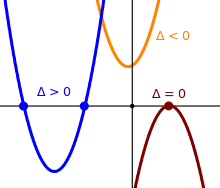# Blog

##### The Discriminant of a Quadratic EquationThe Discriminant of a Quadratic Equation - Part 1

The Discriminant of a Quadratic Equation - Part 2

The Discriminant of a Quadratic Equation - Part 3

The Discriminant of a Quadratic Equation - Part 4

The Discriminant of a Quadratic Equation - Part 5